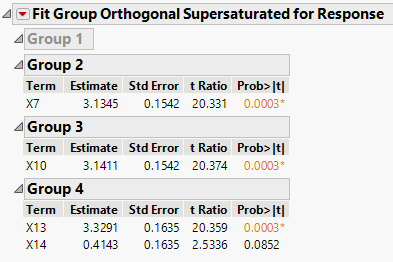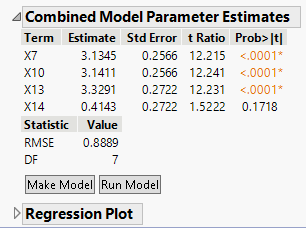Design of Experiments Guide > The Fit Group Orthogonal Supersaturated Platform > The Fit Group Orthogonal Supersaturated Report
Publication date: 03/23/2021

# The Fit Group Orthogonal Supersaturated Report

The Fit Group Orthogonal Supersaturated Report has two sections. The first section reports the estimates of active effects within each group, fitting one group at a time. Only active groups are shown. This is Stage 1 of the analysis. In Stage 2 a combined model is constructed

Figure 27.5 Stage 1 FindingsTerm

Main effects that are identified as active.

Estimate

Parameter estimate for a regression fit of Y on the main effect.

Std Error

The standard error of the estimate, computed using the fake factors in Group 1.

t Ratio

The Estimate divided by its Std Error.

Prob>|t|

The p-value computed using the t Ratio and the degrees of freedom for error (DF).

The second section of the report contains the results of Stage 2 of the analysis. Stage 2 constructs the model used to evaluate the design results.

Figure 27.6 Stage 2 ResultsTerm

Main effects that are identified as active.

Estimate

Parameter estimate for a regression fit of Y on the main effect.

Std Error

The standard error of the estimates obtained by fitting the specified model and intercept using the full data set.

t Ratio

The Estimate divided by its Std Error.

Prob>|t|

The p-value computed using the t Ratio and the degrees of freedom for error (DF).

RMSE

The square root of the mean square error.

DF

The degrees of freedom associated with the error estimate used to construct the RMSE.

Want more information? Have questions? Get answers in the JMP User Community (community.jmp.com).